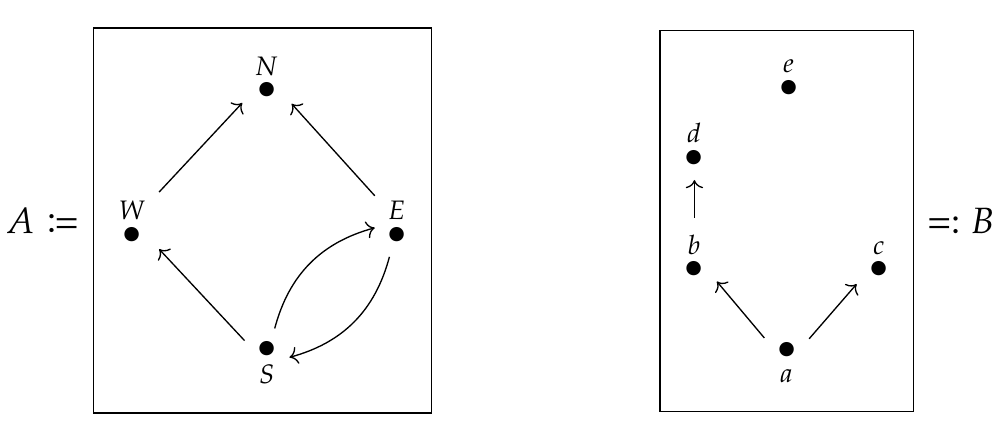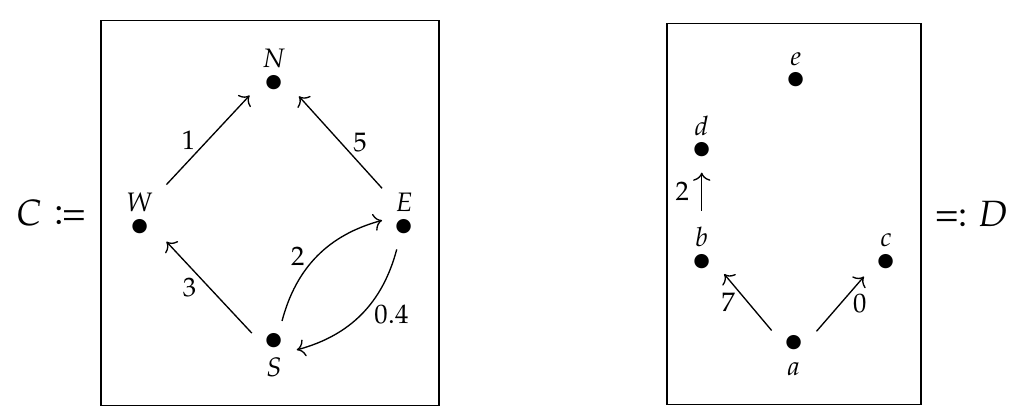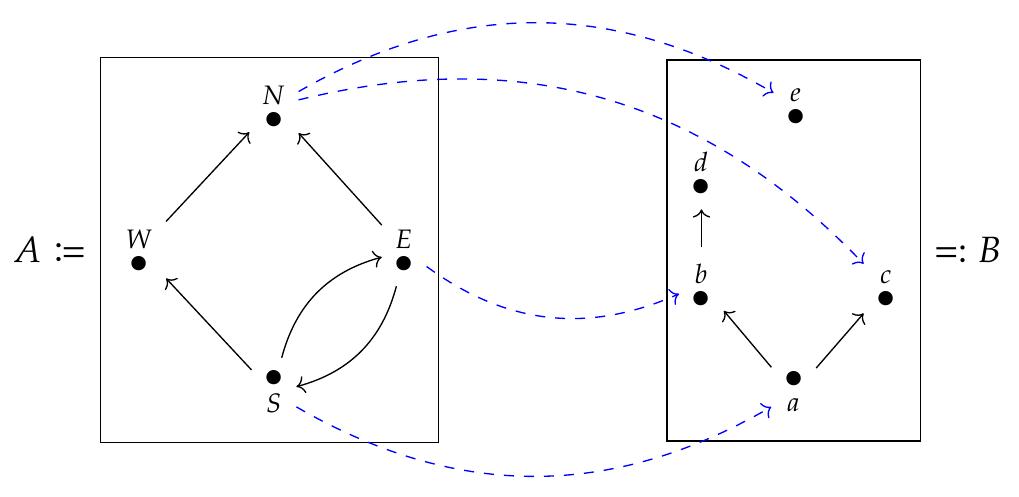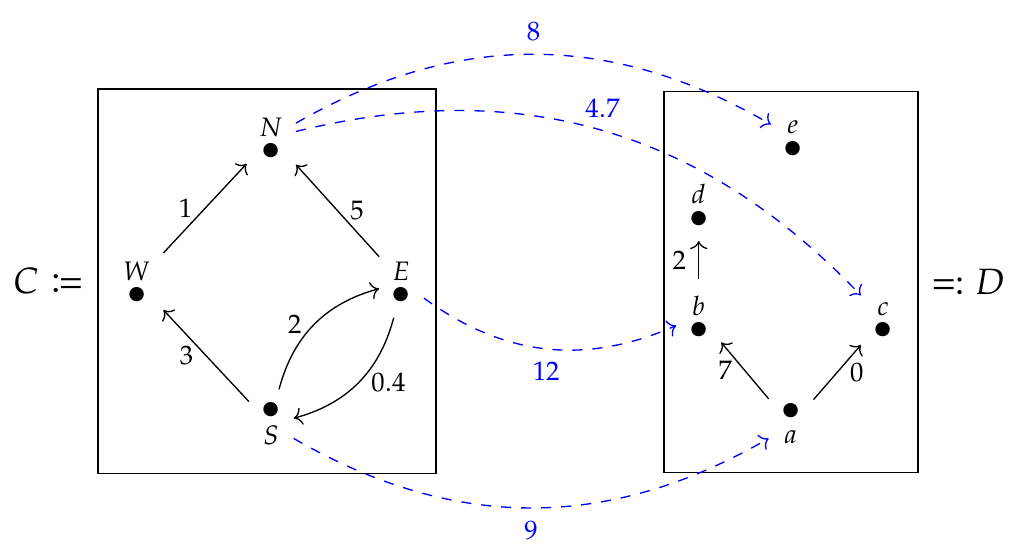We've been looking at feasibility relations, as our first example of enriched profunctors. Now let's look at another example. This combines many ideas we've discussed - but don't worry, I'll review them, and if you forget some definitions just click on the links to earlier lectures!

Remember, \$$\mathbf{Bool} = \lbrace \text{true}, \text{false} \rbrace \$$ is the [preorder](https://forum.azimuthproject.org/discussion/1812/lecture-3-chapter-1-posets/p1) that we use to answer true-or-false questions like

* _can we_ get from here to there?

while \$$\mathbf{Cost} = [0,\infty] \$$ is the preorder that we use to answer quantitative questions like

* _how much does it cost_ to get from here to there?

or

* _how far is it_ from here to there?

In \$$\textbf{Cost}\$$ we use \$$\infty\$$ to mean it's impossible to get from here to there: it plays the same role that \$$\text{false}\$$ does in \$$\textbf{Bool}\$$. And remember, the ordering in \$$\textbf{Cost}\$$ is the _opposite_ of the usual order of numbers! This is good, because it means we have

$\infty \le x \text{ for all } x \in \mathbf{Cost}$

just as we have

$\text{false} \le x \text{ for all } x \in \mathbf{Bool} .$

Now, \$$\mathbf{Bool}\$$ and \$$\mathbf{Cost}\$$ are [monoidal preorders](https://forum.azimuthproject.org/discussion/2082/lecture-21-chapter-2-monoidal-preorders/p1), which are just what we've been using to define [enriched categories](https://forum.azimuthproject.org/discussion/2121/lecture-29-chapter-2-enriched-categories/p1)! This let us define

* [\$$\mathbf{Bool}\$$-enriched categories](https://forum.azimuthproject.org/discussion/2124/lecture-30-chapter-1-preorders-as-enriched-categories/p1), which are just **preorders**

and

* [\$$\mathbf{Cost}\$$-enriched categories](https://forum.azimuthproject.org/discussion/2128/lecture-31-chapter-2-lawvere-metric-spaces/p1), which are **Lawvere metric spaces**.

We can draw preorders using graphs, like these:An edge from \$$x\$$ to \$$y\$$ means \$$x \le y\$$, and we can derive other inequalities from these. Similarly, we can draw Lawvere metric spaces using \$$\mathbf{Cost}\$$-weighted graphs, like these:The distance from \$$x\$$ to \$$y\$$ is the length of the shortest directed path from \$$x\$$ to \$$y\$$, or \$$\infty\$$ if no path exists.

All this is old stuff; now we're thinking about [enriched profunctors](https://forum.azimuthproject.org/discussion/2278/lecture-55-chapter-4-enriched-profunctors/p1) between enriched categories.

A \$$\mathbf{Bool}\$$-enriched profunctor between \$$\mathbf{Bool}\$$-enriched categories also called a [feasibility relation](https://forum.azimuthproject.org/discussion/2281/lecture-57-chapter-4-feasibility-relations/p1) between preorders, and we can draw one like this:What's a \$$\mathbf{Cost}\$$-enriched profunctor between \$$\mathbf{Cost}\$$-enriched categories? It should be no surprise that we can draw one like this:You can think of \$$C\$$ and \$$D\$$ as countries with toll roads between the different cities; then an enriched profunctor \$$\Phi : C \nrightarrow D\$$ gives us the cost of getting from any city \$$c \in C\$$ to any city \$$d \in D\$$. This cost is \$$\Phi(c,d) \in \mathbf{Cost}\$$.

But to specify \$$\Phi\$$, it's enough to specify costs of flights from _some_ cities in \$$C\$$ to _some_ cities in \$$D\$$. That's why we just need to draw a _few_ blue dashed edges labelled with costs. We can use this to work out the cost of going from any city \$$c \in C\$$ to any city \$$d \in D\$$. I hope you can guess how!

**Puzzle 182.** What's \$$\Phi(E,a)\$$?

**Puzzle 183.** What's \$$\Phi(W,c)\$$?

**Puzzle 184.** What's \$$\Phi(E,c)\$$?

Here's a much more challenging puzzle:

**Puzzle 185.** In general, a \$$\mathbf{Cost}\$$-enriched profunctor \$$\Phi : C \nrightarrow D\$$ is defined to be a [\$$\mathbf{Cost}\$$-enriched functor](https://forum.azimuthproject.org/discussion/2169/lecture-32-chapter-2-enriched-functors/p1)

$\Phi : C^{\text{op}} \times D \to \mathbf{Cost}$

This is a function that assigns to any \$$c \in C\$$ and \$$d \in D\$$ a cost \$$\Phi(c,d)\$$. However, for this to be a \$$\mathbf{Cost}\$$-enriched functor we need to make \$$\mathbf{Cost}\$$ into a \$$\mathbf{Cost}\$$-enriched category! We do this by saying that \$$\mathbf{Cost}(x,y)\$$ equals \$$y - x\$$ if \$$y \ge x \$$, and \$$0\$$ otherwise. We must also make \$$C^{\text{op}} \times D\$$ into a \$$\mathbf{Cost}\$$-enriched category, which I'll let you figure out to do. Then \$$\Phi\$$ must obey some rules to be a \$$\mathbf{Cost}\$$-enriched functor. What are these rules? What do they mean concretely in terms of trips between cities?

And here are some easier ones:

**Puzzle 186.** Are the graphs we used above to describe the preorders \$$A\$$ and \$$B\$$ [Hasse diagrams](https://en.wikipedia.org/wiki/Hasse_diagram)? Why or why not?

**Puzzle 187.** I said that \$$\infty\$$ plays the same role in \$$\textbf{Cost}\$$ that \$$\text{false}\$$ does in \$$\textbf{Bool}\$$. What exactly is this role?

By the way, people often say **\$$\mathcal{V}\$$-category** to mean \$$\mathcal{V}\$$-enriched category, and **\$$\mathcal{V}\$$-functor** to mean \$$\mathcal{V}\$$-enriched functor, and **\$$\mathcal{V}\$$-profunctor** to mean \$$\mathcal{V}\$$-enriched profunctor. This helps you talk faster and do more math per hour.

**[To read other lectures go here.](http://www.azimuthproject.org/azimuth/show/Applied+Category+Theory#Chapter_4)**